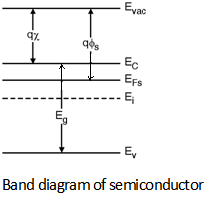Home > Digital CMOS Design > MOS Capacitor > MOS Definitions

CMOS-Inverter

MOS Definitions : For a MOS junction two important parameters are the electron affinity and the work function.

Figure shows the band diagram of metal.Further, Figure below shows the band diagram of semiconductor. In these figures the Eg is the band gap energy of the semiconductror, EFm and EFs are the fermi energy of the metal and semiconductor respectively, and Ei is the intrinsic energy level of the semiconductor.We also define a new energy level in these band diagrams which is denoted by the vaccume level (Evac).

For a semiconductor the energy required to excite an electron from its conduction band EC to the vaccume level Evac is known as the electron affinity (qχ) of the semiconductor. For a given semiconductor, it has a fixed value and for silicon its typical range is between 4.05 eV to 4.15 eV at room temperature.

Further, the difference in energy levels of fermi level and the vaccume is called as work function (qΦm ).

Furthermore, the difference in energy level in fermi level of semiconductor and the vaccume level is called as semiconductor work function (qΦs).